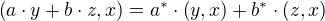A Hilbert Space is generalisation of euclidean space.

A Hilbert Space H is an Inner Product Space that has an induced Distance Function (Norm) like this:(with x∈H)

Since H is Inner Product Space, it holds that:swapping of inner product operands is complex conjugation of the resultLinearity in the second argumentpositive definiteness (only when both arguments are the same)Antilinearity in the first argumentCauchy-Schwarz inequalityAbsolute Convergence of all vectors

## Dirac Hilbert Space

In Quantum Mechanics, a specific Hilbert Space of Square Integrable Functions L² is used:Note that the above, in general, is a complex numberThis is a real number.

A vector in this space is written as:Ket Vector

A Hilbert Space is a linear vector space, so:CommutativityAssociativityScalar multiplicationDistributivity

The Hilbert Space is complete, so every linear combination of vectors in a Hilbert space is again a vector in the same Hilbert space.

The Bra Vector is the complex conjugate of the Ket Vector, an element of the dual space of H, also an element of the same Hilbert space H:Reversal of Operators

Reason for the reversal of operators:Definition of Adjoint Operator L^† to L

### Components

Letbe a (for example orthonormal) basis. Then because of the completeness, any vectorcan be written as a linear combination with respect to that basis:Then the coefficients can be determined by completing the scalar product over the equation, just as one would within the Euclidean SpaceIf the basis is orthonormal, it follows thatWe say the projection operator P is (without sum):Projection is idempotent, projecting multiple times in a row does not change the result.

On the other hand, we say that the identity operator is:By applying this operator both to the left and to the right side of another operator L, we get its components with respect to a basis:are the components with respect to the basis ψ_....